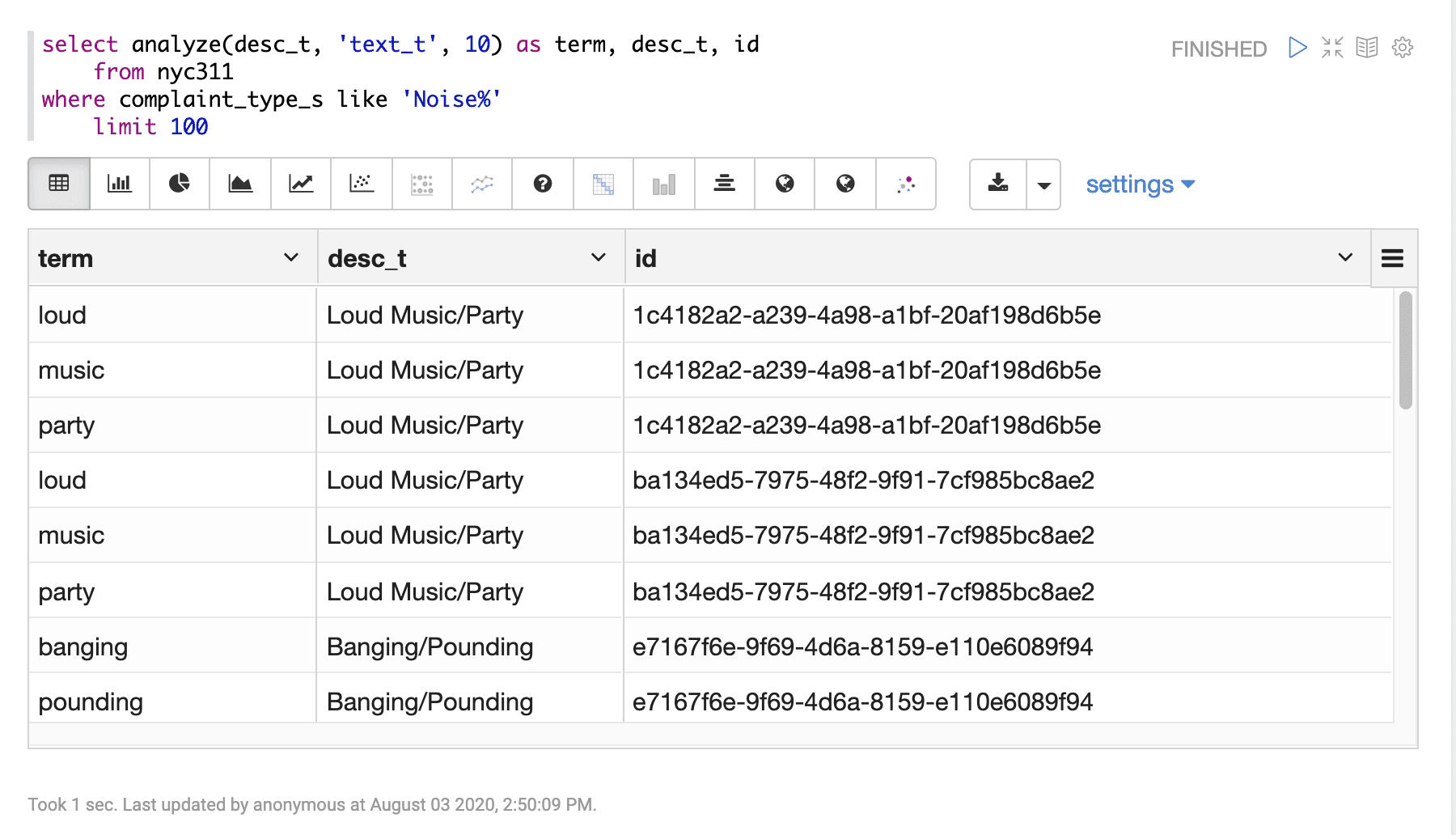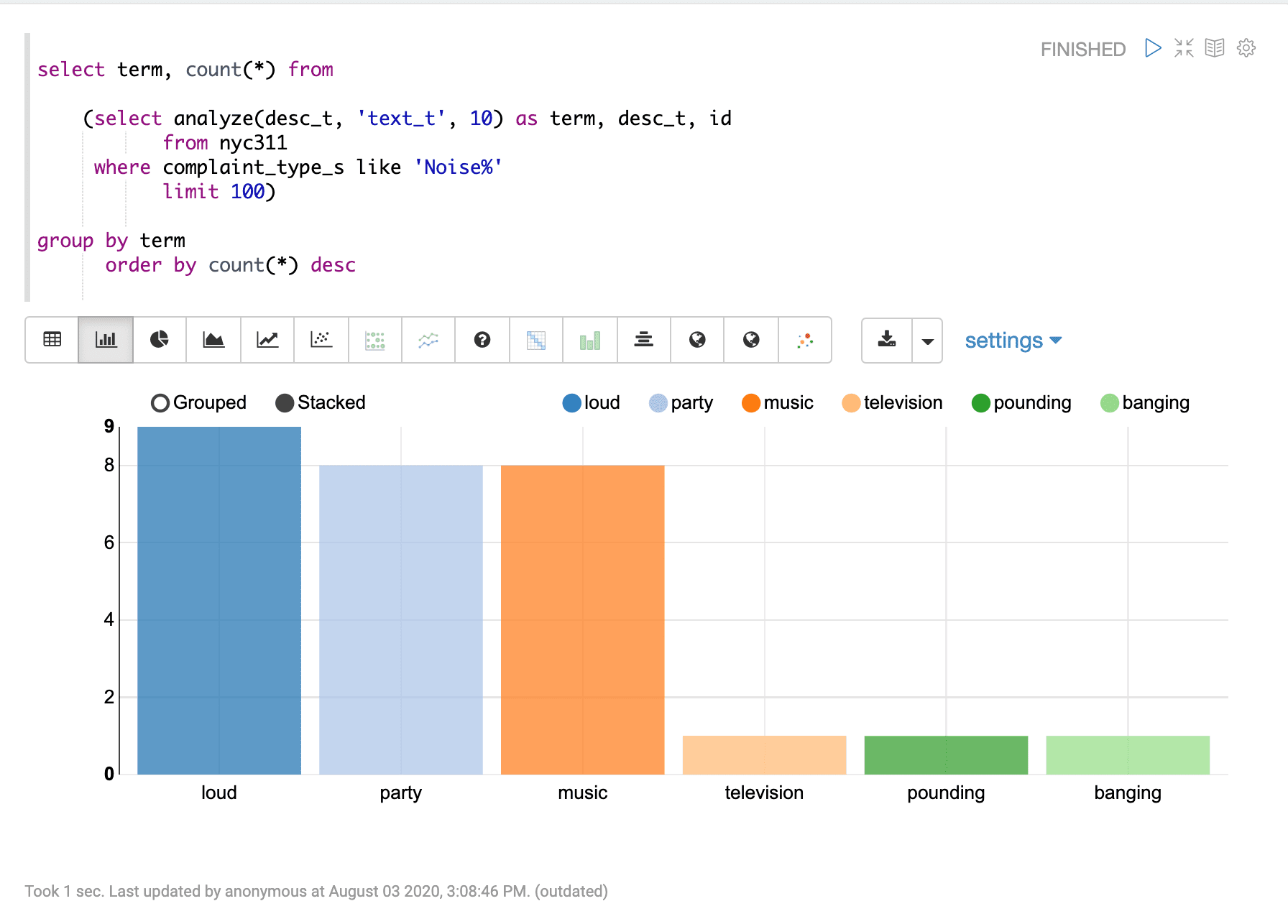# Text Analysis and Analytics (analyze)

The `analyze` function analyzes a text or string field with a Lucene/Solr analyzer and returns the analyzed tokens. The use of Lucene/Solr analyzers at query time allows for real-time entity extraction by configuring OpenNLP, Stanford NLP or other NLP analyzers.

The `analyze` function takes three parameters:

1. the text or string field to analyze

2. a string literal pointer to the Lucene/Solr analyzer to use

This can be either a field name in the Solr schema with an analyzer defined, or a string that matches a dynamic field pattern.

3. the number of documents to analyze

Which documents are analyzed is determined by the `WHERE` clause. The documents are ordered by score descending to determine the top N documents to analyze.

## Sample syntax

``````select analyze(desc_t, 'text_t', 10) as term,
desc_t,
id
from nyc311
where complaint_type_s like 'Noise%'
limit 100``````

## Result set

The analyzed result set contains a row for each analyzed term. The `analyze` function returns the term. Other fields from the record can be output with the term as well. The number of terms returned in the result set is controlled by the LIMIT clause.

Sample result set in Apache Zeppelin:## Text analytics

A SQL aggregation query can be wrapped around the analyzed result set to perform aggregation over the terms. Below is an example of the wrapped aggregation query:

``````select term, count(*) from

(select analyze(desc_t, 'text_t', 10) as term, desc_t, id
from nyc311
where complaint_type_s like 'Noise%'
limit 100)

group by term
order by count(*) desc``````

## Visualization

The aggregations over the analyzed result set can be visualized as bar or pie charts. The example below shows the aggregations visualized as a bar chart: